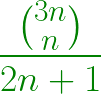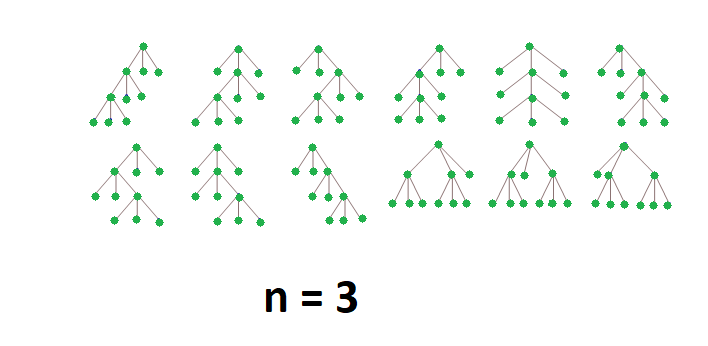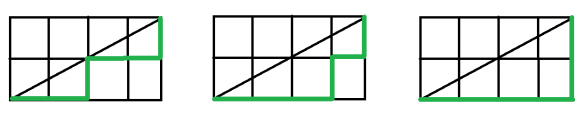Program for nth Fuss–Catalan Number

• Last Updated : 06 Apr, 2021

Fuss–Catalan Numbers are a generalization of Catalan numbers that uses triplets instead of pairs.

The Fuss-Catalan Numbers can be represented by a Series with the formula:The first few Fuss–Catalan Numbers are

1, 1, 3, 12, 55, 273, 1428, 7752, 43263, 246675………..
for n = 0, 1, 2, 3, … respectively

Applications of Fuss-Catalan number:

1. Count the number of ways to place parentheses among of 2n+1 numbers to be grouped three at a time.
Example: There are 3 ways to parenthesize {1, 2, 3, 4, 5} as triplets:
{{1, 2, 3}, 4, 5}, {1, {2, 3, 4}, 5}, {1, 2, {3, 4, 5}}

2. Count the number of complete ternary trees with n internal nodes.1.
2. Count the number of paths of length 3n through a 2n-by-n grid that does not cross above the main diagonal
Example: There are 3 paths from (0, 0) to (4, 2) that don’t cross above the diagonal:1.

Implementation of Fuss-Catalan number:

C++

 // C++ program for nth Fuss–Catalan Number #include using namespace std; // Returns value of Binomial Coefficient C(n, k)unsigned long int binomialCoeff(unsigned int n,                                unsigned int k){    unsigned long int res = 1;     // Since C(n, k) = C(n, n-k)    if (k > n - k)        k = n - k;     // Calculate value of    //[n*(n-1)*---*(n-k+1)] / [k*(k-1)*---*1]    for (int i = 0; i < k; ++i) {        res *= (n - i);        res /= (i + 1);    }     return res;} // A Binomial coefficient based function// to find nth Fuss–Catalan number in O(n) timeunsigned long int Fuss_catalan(unsigned int n){    // Calculate value of 3nCn    unsigned long int c = binomialCoeff(3 * n, n);     // return 3nCn/(2n+1)    return c / (2 * n + 1);} // Driver codeint main(){    for (int i = 0; i < 10; i++)        cout << Fuss_catalan(i) << " ";    return 0;}

Java

 // Java program for nth Fuss-Catalan Numberclass GFG{ // Returns value of Binomial Coefficient C(n, k)static int binomialCoeff(int n, int k){    int res = 1;     // Since C(n, k) = C(n, n-k)    if (k > n - k)        k = n - k;     // Calculate value of    //[n*(n-1)*---*(n-k+1)] / [k*(k-1)*---*1]    for (int i = 0; i < k; ++i)    {        res *= (n - i);        res /= (i + 1);    }    return res;} // A Binomial coefficient based function// to find nth Fuss-Catalan number in O(n) timestatic int Fuss_catalan(int n){    // Calculate value of 3nCn    int c = binomialCoeff(3 * n, n);     // return 3nCn/(2n+1)    return c / (2 * n + 1);} // Driver codepublic static void main(String []args){    for (int i = 0; i < 10; i++)        System.out.print(Fuss_catalan(i) + " ");}} // This code is contributed by 29AjayKumar

Python3

 # Python3 program for nth Fuss–Catalan Number # Returns value of Binomial Coefficient C(n, k)def binomialCoeff(n, k) :     res = 1;     # Since C(n, k) = C(n, n-k)    if (k > n - k) :        k = n - k;     # Calculate value of    # [n*(n-1)*---*(n-k+1)] / [k*(k-1)*---*1]    for i in range(k) :                 res *= (n - i);        res //= (i + 1);     return res; # A Binomial coefficient based function# to find nth Fuss–Catalan number in O(n) timedef Fuss_catalan(n) :     # Calculate value of 3nCn    c = binomialCoeff(3 * n, n);         # return 3nCn/(2n+1)    return c // (2 * n + 1); # Driver codeif __name__ == "__main__" :     for i in range(10) :        print(Fuss_catalan(i), end = " "); # This code is contributed by AnkitRai01

C#

 // C# program for nth Fuss-Catalan Numberusing System; class GFG{ // Returns value of Binomial Coefficient C(n, k)static int binomialCoeff(int n, int k){    int res = 1;     // Since C(n, k) = C(n, n-k)    if (k > n - k)        k = n - k;     // Calculate value of    //[n*(n-1)*---*(n-k+1)] / [k*(k-1)*---*1]    for (int i = 0; i < k; ++i)    {        res *= (n - i);        res /= (i + 1);    }    return res;} // A Binomial coefficient based function// to find nth Fuss-Catalan number in O(n) timestatic int Fuss_catalan(int n){    // Calculate value of 3nCn    int c = binomialCoeff(3 * n, n);     // return 3nCn/(2n+1)    return c / (2 * n + 1);} // Driver codepublic static void Main(String []args){    for (int i = 0; i < 10; i++)        Console.Write(Fuss_catalan(i) + " ");}} // This code is contributed by PrinciRaj1992

Javascript


Output:
1 1 3 12 55 273 1428 7752 43263 246675

Time Complexity: O(n)

My Personal Notes arrow_drop_up